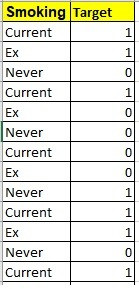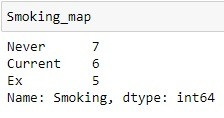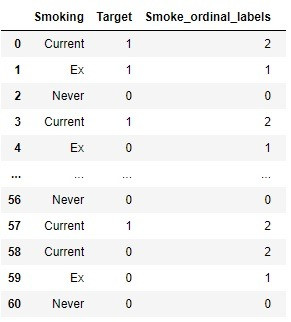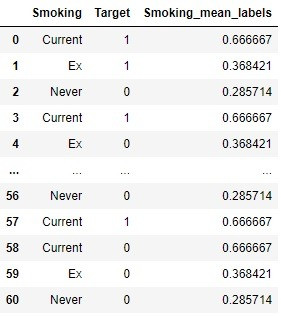Search

# Handling Categorical Data in Machine Learning through Python

Everyone needs an environment in life which is comfortable and in which one can adapt.

You cannot live in an alien world and interact.

As computer has its own language, machine learning algorithms work on numerical data. This blog is about what we can do when there is categorical data in the dataset. How to handle it and make it useful for the machine learning algorithm to get insightful information.

We are taking an example of a simple data, about smoking status and target which Smoking state and lung problem.There are different ways of getting information out of categorical features suitable for using statistical tools in machine learning algorithms.

Few of the ways are as under:

1. One hot Encoding

One-Hot Encoding is a very handy and popular technique for treating categorical features. This is based on creating additional features by its unique values. Every unique value in this is a added feature and values are assigned as 1 or 0 based on the presence of it in a row.

In Python it can be implemented as:

```list=data.Smoking.value_counts().sort_values(ascending=False).index list=list(list)
import numpy as np
for categories in list:
data[categories]=np.where(data[‘Smoking']==categories,1,0)```

Output:Assumptions:

1. There are finite set of features.

2. Where no ordinal relationship exists between the categories of variable.

Advantages:

1) Easy to use.

2) Creates no bias as assumption of any ordering between the categories.

Disadvantages:

1) Can result in an increase in number of features resulting in performance issues.

2. Ordinal number Encoding

This is a popular technique, in which each label is assigned a unique integer based on alphabetical ordering.

This is easiest way and used in most of the data where there is natural relation between the categories of ordinal values.

In Python it can be done as:

```# Import label encoder
from sklearn import preprocessing
# label_encoder object knows how to understand   word labels.
label_encoder = preprocessing.LabelEncoder()
# Encode labels in column 'Smoking'.
data[‘Smoking_Ordinal’]= label_encoder.fit_transform(data[‘Smoking’])
print(data.head())```

Or

```dictionary={'Current': 0, 'Ex': 1, 'Never': 2}
data['Smoking_ordinal']=data[‘Smoking’].map(dictionary)```

Output:Assumptions:

The integer values are having natural ordered relationship between each other. Like Current>Ex>Never.

Advantages:

1) Easy to use

2) Easily reversible.

3) Doesn't increase feature space .

Disadvantages:

1) May result in unexpected results if the ordering of number is not related in any order.

3. Count or Frequency Encoding

In this type of encoding the count of existence of each category in the variable is determined. Each category is then replaced by the frequency of it.

In Python it can be implemented as:

finding the count

`Smoking_map=data1['Smoking'].value_counts()`

Output:count is stored in dictionary and mapped to category.

```Smoking_map=data1['Smoking'].value_counts().to_dict()
data1['Smoking_Freq']=data1['Smoking'].map(Smoking_map)```

Output:Assumptions:

There is no category of variable having similar frequency.

Advantages

• Easy to use.

• Easily reversible.

• Doesn't increase feature space .

Disadvantages

• Will not be able to handle if frequencies are same for two or more categories.

#### 4. Ordinal Encoding As per Target

In this type of encoding categories of a feature are replaced as per the target feature, i.e as per the sorting order of maximum true in target for a category.

Ordering the categories as per target.

In Python it can be implemented as:

```mean=data1.groupby(['Smoking'])['Target'].mean()
Ord_Labels=mean.sort_values().index
ordinal_labels={k:i for i,k in enumerate(Ord_Labels,0)}

data1['Smoke_ordinal_labels']=data1['Smoking'].map(ordinal_labels)```

`mean`

Smoking Current 0.666667 Ex 0.368421 Never 0.285714 Name: Target, dtype: float64

`ordinal_labels`

{'Never': 0, 'Ex': 1, 'Current': 2}

i.e. as we can see 'Current' is maximum hence more value is assigned to it, followed by 'Ex' and 'Never'.

And as per this the labels are updated.

Output:Assumptions:

High cardinality categorical features.

Advantages:

1) It makes a monotonic relationship with target.

Disadvantages:

1) May cause overfitting.

#### For each category mean is calculated as per target and the same value is assigned

In Python it can be implemented as:

```dict_mean=data1.groupby(['Smoking'])['Target'].mean().to_dict()
data1['Smoking_mean_labels']=data1['Smoking'].map(dict_mean)```

`dict_mean`

Smoking Current 0.666667 Ex 0.368421 Never 0.285714

Output:Assumptions:

High cardinality categorical features.

Advantages:

1) It makes a monotonic relationship with target.

2) Doesn't affect the volume of the data and helps in learning faster.

Disadvantages:

1) Model may overfit

2) Information can be lost if categories are divided into very few categories because of their density.

3) Hard to validate the results.

4) Fewer splits, faster learning.

I hope by using these simple encoding techniques most of categorical categories could be handled.

There are more encoding techniques to discuss, till then, happy data handling.

Thanks for reading!

195 views0 comments

### Recent Posts

See All

#### API/Web Service Overview:

So lets start off by learning what exactly is a Web Service? Its a method of communication between two applications or electronic devices over the worldwide web. Here is an example: Consider a flight

#### Application Programming Interface…Messenger or an IT terminology?

Being from a non-IT background, I always try to make IT terms simpler and more comprehensible. So, beginning with the question what is an API? How the internet works behind the scenes. We use our lapt